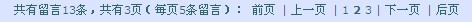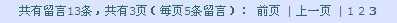### #1 jake

`Array(    [total_count] => 13  // 数据总记录    [page_size] => 5    // 每页多少条记录        [total_page] => 3    // 总页数    [first_page] => 1     // 第一页的页码    [prev_page] => 1     // 上一页的页码    [next_page] => 2     // 下一页的页码    [last_page] => 3      // 最后一页的页码    [current_page] => 1   // 当前页码    [all_pages] => Array   // 页码数据，这里是全部的页码        (             => 1             => 2             => 3        ))`

`<{if \$pager}>共有留言<{\$pager.total_count}>条，共有<{\$pager.total_page}>页（每页<{\$pager.page_size}>条留言）：<{if \$pager.current_page != \$pager.first_page}>前页 | 上一页 | <{/if}><{foreach from=\$pager.all_pages item=thepage}>        <{if \$thepage != \$pager.current_page}>                <{\$thepage}>        <{else}>                <{\$thepage}>        <{/if}><{/foreach}><{if \$pager.current_page != \$pager.last_page}> | 下一页 | 后页<{/if}><{/if}>``<{if \$pager}>`

`共有留言<{\$pager.total_count}>条，共有<{\$pager.total_page}>页（每页<{\$pager.page_size}>条留言）：``<{foreach from=\$pager.all_pages item=thepage}>        <{if \$thepage != \$pager.current_page}>                <{\$thepage}>        <{else}>                <{\$thepage}>        <{/if}><{/foreach}>`2012-08-04 16:39:23